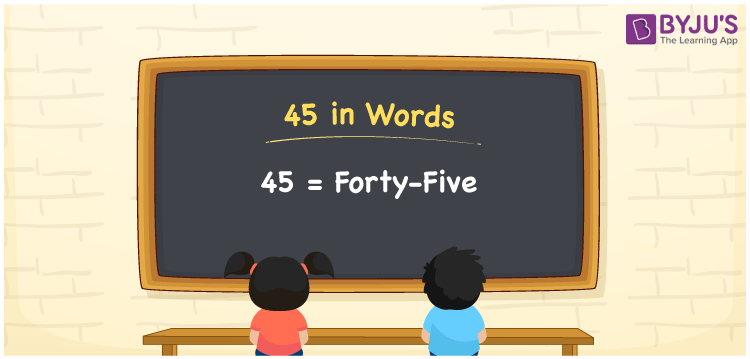# 45 in Words

45 in words is written as “Forty-five”. 45 is 5 more than 40. In the number system, 45 is a number that represents a value or a count equivalent to it. For example, Arsh has bought a book for Rs.45. Using words, we can write the same sentence as Arsh has bought a book for Rupees Forty-five. Thus, we can have many real-life examples where the numbers are presented in words. Thus it is necessary for students to learn the number names to read, write and express them. Let us learn how to write 45 in words in this article.

 45 in words Forty-five Forty-five in Numbers 45

## 45 in English Words

We generally write numbers in words using the English alphabet. Thus, we can read 45 in English as “Forty-five”.## How to Write 45 in Words?

To convert 45 in words, we need to check the place value of its digits. Since 45 is a two-digit number, thus, place value of 4 is tens and 5 is ones. The order of place values of digits in a number is in accordance with the Indian numbering system, such as:

• Ones
• Tens
• Hundreds
• Thousands
• Ten-thousands
• Hundred-thousands or Lakhs

This can be done as follows.

 Tens Ones 4 5

This can be written as:

4 × Ten + 5 × One

= 4 × 10 + 5 × 1

= 40 + 5

= 45

= Forty-five

Thus, 45 in words is written as Forty-five.

45 is a natural number that precedes 46 and succeeds 44.

45 in words – Forty-five

Is 45 an odd number? – Yes

Is 45 an even number? – No

Is 45 a perfect square number? – No

Is 45 a perfect cube number? – No

Is 45 a prime number? – No

Is 45 a composite number? – Yes

## Frequently Asked Questions on 45 in Words

Q1

### How do you write 45 in English?

We can write 45 in English words as “Forty-five”.
Q2

### What is 145 in English?

145 is written as One hundred and forty-five in English.
Q3

### Is 45 an even number or an odd number?

45 is an odd number since it is not completely divisible by 2.